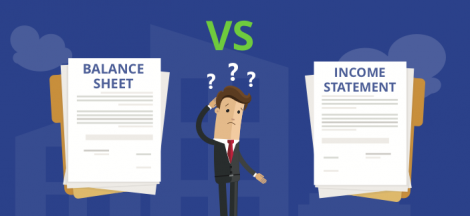Data analysis is the process of using data to derive knowledge and understanding. It is a critical component of many decision-making processes, including business analysis, marketing research, and public health analysis.

Data analysis is one of the most important aspects of any business. It is the process of sorting, organizing, and summarizing data so that it can be used to make informed decisions. This information can then be used to improve the operations of the business, attract new customers, and forecast future trends.

When done correctly, data analysis can provide insights that allow businesses to make better decisions and improve their bottom line. By understanding customer preferences, for example, a business can tailor its offerings to better meet the needs of its customers.

In addition, data analysis can help businesses to identify potential problems and to develop solutions. By monitoring customer trends, for example, a business can detect patterns that might indicate a problem before it becomes serious.

Moreso, data analysis is essential to the success of any business. It can help to identify trends, make informed decisions, and improve the overall operation of the business.

Types of Data Analysis

There are a number of different types of data analysis that can be used to gain insights into a given situation or problem. Data analysis can be descriptive, inferential, and regression in nature.

Descriptive analysis is used to provide a broad overview of the data. It can be used to describe the size, structure, and demographics of the population being analyzed.

Inference statistical analysis is used to draw conclusions about the data. It can be used to identify relationships between variables, assess statistical significance, and make predictions.

Regression analysis is used to assess the relationship between two or more variables. It can be used to identify causes and effects of changes in the data.

One of the most common types of data analysis is statistical analysis. Statistical analysis involves using data to identify patterns and trends, and can be used to generate hypotheses about how the data might be related to one another. Statisticians use a variety of statistical techniques to carry out statistical analysis, including descriptive statistics, inferential statistics, and regression analysis.

Another common type of data analysis is anecdotal data. An anecdotal data analysis is based on observations made about a given situation, and can be used to generate hypotheses about how the data might be related to one another. An anecdotal data analyst may collect data from interviews, observation, or surveys.

Finally, data visualization can be used to help understand and understand data.

Data visualization in probability

Probability is the measure of the likelihood that something will happen. Probability can be thought of as the chance of an event happening. Probability is always a two-sided coin – there are both positive and negative probabilities. Probability is always expressed as a percentage. For example, the probability of throwing a fair coin is 50%. This means that there is a 50% chance that the coin will land on heads and a 50% chance that the coin will land on tails.

Probability is used in a lot of different areas of life. For example, Probability is used to calculate the odds of winning a lottery. Probability is also used to calculate the chances of getting a particular result in a game of chance, such as roulette.

Probability is also used in the field of statistics. Statistics is the study of patterns and trends. Statistics is used to figure out how likely it is that a particular event will happen. For example, statistics is used to figure. #MMBA3## Understanding the Balance Sheet and the Income Statement: A Guide for Business Managers #MMBA5

in